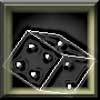#### You may also like### Gambling at Monte Carlo

A man went to Monte Carlo to try and make his fortune. Is his strategy a winning one?### Marbles and Bags

Two bags contain different numbers of red and blue marbles. A marble is removed from one of the bags. The marble is blue. What is the probability that it was removed from bag A?### Coin Tossing Games

You and I play a game involving successive throws of a fair coin. Suppose I pick HH and you pick TH. The coin is thrown repeatedly until we see either two heads in a row (I win) or a tail followed by a head (you win). What is the probability that you win?

# Distribution Differences

### Why do this problem:

When we compare data sets we use various measures and descriptions. This problem invites students to explore a similar question : how can probability distributions be compared?

### Possible approach :

Practical work seems particularly important for this problem.

Start with estimating 10 cm. Give the group a minute or two to practise with a ruler, then with all measures or samples out of sight ask them to put two marks on a fresh piece of paper. The data are collected, to the nearest mm. Before examining the data invite students to make a guessed description of the data set. Is it symmetric ? What is it centred on? How dispersed?

Next take five dice and run 20 trials counting the number of sixes each time. Ask students to plot that frequency distribution.

If possible have each member of the group with a shuffled pack of cards. Conduct the synchronised card turning and collect the number of Aces of Spades observed at each card turn. If the group is small perhaps report every ace, and later ask what effect this change in the rules had on the distribution. Similarly any royal card might also be included in the set of cards reported. As before ask the group how this affects the distribution.

Now work with tossed coins. Five, as with the dice, and also one coin for every member of the group. This should help students see how the dice and cards activities are structurally the same. They differ from the coin tossing in their asymmetry and it can be seen how both the chance of a sighting (six or ace) and the sample size (five dice or hundred packs of cards) affect the distribution.

Although the vocabulary 'discrete' and 'continuous' may help distinguish these two from the distribution of 10cm estimates, acquisition of correct technical terms is not the most important benefit.

There is plenty to discuss about the 10cm estimates: Is this variable random? Is it symmetric? Does this data sample match that?

One key point to include within the discussion is that once we collect the data to the nearest mm it becomes discrete but the variable itself was continuous (there are no two distinct values which cannot have another value between them)

### Key questions :

• How do we compare two sets of data, say for height statistics for 11 year olds now and fifty years ago? Why can't we do exactly the same with probability and sample data?

• When five dice are rolled what is the probability that we see no sixes, or one six only, or two sixes, three, four, or five? What will the probability values for each of these come to as a total?

• When a person estimates 10cm do you think there is probably more chance of their estimate being within one centimetre of 10cm than of being off by between 5cm and 6cm? Do you think estimating too much is as likely as too little? Why?

• How is Aces High like Five Dice? How is it different? And what if this involved tossing a coin rather than dice and cards?

• 10cm is different to Five Dice in some key ways - what would you say those differences were?

### Possible extension :

The Data Matching challenge will develop this theme of similarity and difference between sample sets from random variables.

### Possible support :

One area to build up for less able students could be the presence or absence of symmetry in the sample and in the theoretical distribution. Students can count sixes on three dice and count heads from three coins. There is also value in comparing two dice where the variable of interest is the sum and two dice where the variable is the number of sixes to help students understand that it isn't dice that create symmetry or asymmetry but the particular variable in which we take an interest.